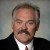# Can you explain "degrees of freedom" and provide an example?

ByDr. Mikel Harry

To facilitate an answer, we will consider three examples.  First, consider the game of baseball.  We understand the field-of-play consists of 9 positions.  The coach is “free” to assign any of the 9 players to any of the 9 positions. Once the 8th player is assigned to the 8th position, the 9th player-position is pre-determined, so to speak.  In other words, the coach is not “free” to pick either the last position or the last player.

For every position that is assigned, the remaining number of choices (decision options) is decreased by one.  In other words, one degree-of-freedom (decision option) is lost every time a position-player decision is made.  When considering the last position (and player) there is no choice of selection (or assignment) – simply because the total number of positions and players is fixed in advanced (by virtue of the game’s rules).

Let us now consider a second analogy.  Suppose we have before us a simple wire with some beads on it.  Of course, we are talking about something like coat hanger wire and costume jewelry beads.  So as to work with some “hard numbers,” it will be known that there is only 1 wire rod and 8 beads, where each bead has a small hole in the middle.

Now, imagine that we thread each of the 8 beads onto the wire rod.  Further imagine that this is done in such a way that the beads are “free” to move anywhere on the rod, but without falling off.  For the sake of discussion, it will be known that the goal is to suspend or otherwise hold the rod of beads in space (parallel to the ground).  To do this, we will assert that the left-hand must grasp a bead, as well as the right-hand.  Only in this manner (by definition), can the “rod of beads” be properly suspended in space.

Handpicked Content:   How does normality of the data impact the process performance?

Given these restrictions, it should now be apparent, that if we grasp any two of the beads, so as to meet the goal, then N –2 = 8 – 2 = 6 of the beads would be “fee to vary” somewhere along the rod, but 2 of the 8 could not vary (as they are used to fix the rod in space).  Thus, for the given scenario, we have N – 2 = 6 degrees-of-freedom.

As a final analogy let us say that there exists four independent categories related to some phenomenon.  Say categories A, B, C, and D, respectively.  Now let us postulate that we previously studied the four categories and made a total of 10 observations.  It is known that, of the 10 categorical observations, 2 were assigned to category “A,” 3 were assigned to category “B,” and 1 was assigned to category “C.”  However, let us say that we somehow lost all of the observations related to category “D.”  In this case, we don’t know how many type “D” observations were made.  Well, this would not be a problem because of three things.

First, we know the total number of observations that were made (N = 10).  Second, we know that N = A + B + C + D.  Third, we know that the exact number of observations assigned to the other three categories (A = 2, B = 3, and C = 1).  Thus, it can be reasoned that N – D = A + B + C.  Solving for D, we note that D = N – (A + B + C), or D = 10 – ( 2 + 3 + 1) = 10 – 6 = 4.  Since the total number of categorical observations was known in advance, and the other three categorical quantities were also known, then the “lost” categorical quantity can be determined by algebraic solution – its value is “not free to vary.”  Hence, the degrees-of-freedom (df) would be expressed as df = c –1 = 4 – 1 = 3, where c is the total number of categories.

##### Dr. Mikel Harry

1.pushpa

Very good explanation, Thank you so much!!

2.Thank you so much. I’d like to use your baseball example in my classes (if you don’t mind). I think the definition of df will be readily apparent to my undergraduates.

3.Dr. Moataz

Thanks a lot

4.varun

nice… i got d examples … and i got a few statements..bt how can it repel on it,,????

5.Shonell Hinds

Thanks this was very clear and most helpful. Appreciated!

6.Jen Cogliano

I just want to tell the person that wrote this that this is the best explanation of df I’ve come across. It was very helpful in clarifying what df is.
Thank you